Next: Parameter Estimation for Up: Gaussian Markov Random Previous: Iterative Gaussian Markov

### Direct Gaussian Markov Random Field Sampler

The previous section outlined an algorithm for sampling GMRF textured images using an iterative method. Unfortunately, this algorithm may have to perform hundreds or even thousands of iterations before a stable texture is realized. Next we present a scheme which makes use of two-dimensional Fourier transforms and does not need to iterate. The Direct GMRF Sampler algorithm is realized from  as follows. We use the following scheme to reconstruct a texture from its parameters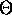and a neighborhood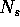: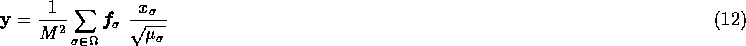where y is the resulting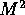array of the texture image, and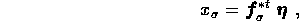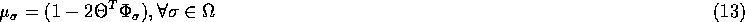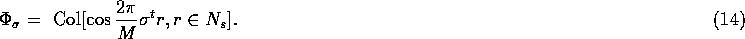The sampling process is as follows. We begin with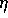, a Gaussian zero mean noise vector with identity covariance matrix. We generate its the Fourier series, via the Fast Fourier Transform from Subsection 2.4, using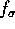, the Fourier vector defined below: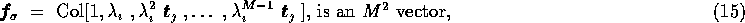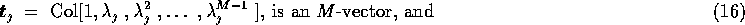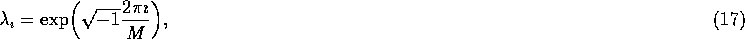and finally apply (12).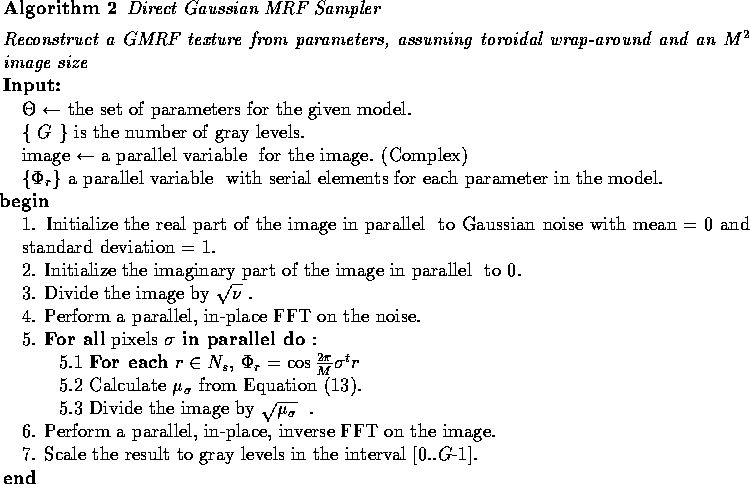Steps 1, 2, 3, 5.2, 5.3, and 7 all run in O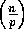parallel steps, where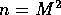and p is the number of processors available. As stated in Subsection 2.4, an n-point FFT, used in steps 4 and 6, computed on p processors takes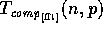computation time and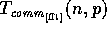communications. Step 5.1 takes O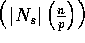parallel steps.

The Direct Gaussian MRF Sampler algorithm thus has a computation complexity of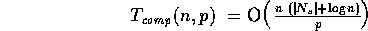and communication complexity of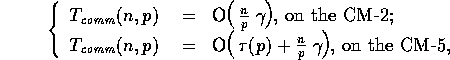using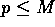processors.

Note that the number of gray levels, G, is only used in the last step of the algorithm as a scaling constant. Hence this algorithm scales with image size n and number of processors p, independent of the number of gray levels G used. Notice also that the communication complexity is higher than that of the Gibbs sampler; this is due to the fact that the FFT is a global operation on the image. Our experimental data collected by implementing this algorithm on the CM-2 and the CM-5 confirm our analysis.Next: Parameter Estimation for Up: Gaussian Markov Random Previous: Iterative Gaussian Markov